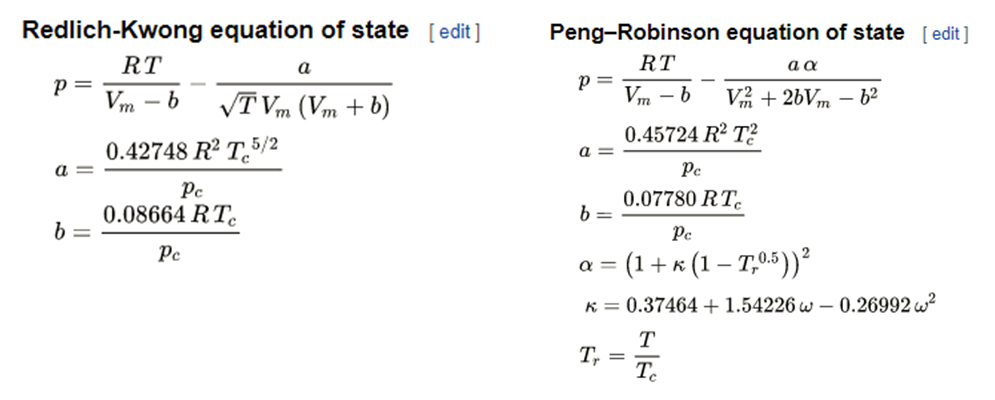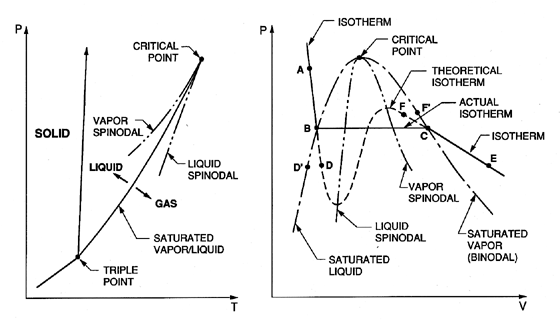Last time we discussed the ideal early days of thermophysical properties. Specifically we looked into the progression of the ideal gas law by the natural philosophers and eventually physicists that culminated in the virial equation of state in 1905. After this, physicists generally took the research into the micro-scale and using statistical thermodynamics to show how quantum phenomena affect bulk properties. Over the years many intermolecular potential assumptions have been mapped to the virial equation to model bulk properties — hard-sphere potentials, soft-sphere potentials, squishy-sphere potentials, staticky-sphere potentials, non-spherical potentials, etc. Even going to direct solution of the Schrodinger wave equation to determine the potentials. Aside from direct Schrodinger wave solution, which is still computationally prohibitive for most systems, all of these approaches very much remain approximations, approximations that ultimately struggled to model real condensed fluid behavior, specifically phase change. These not so ideal later days of thermophysical properties are when the engineers took over — for awhile at least.

###### Phase change and the Cubic Equations of State

In the 1840’s shortly after Clapeyron developed his equation for ideal-gas behavior, Thomas Andrews, an Irish Scientist, conducted a complete review of the equation while studying the behavior of carbon dioxide. Andrews was the first to study the Pressure*Volume behavior of fluids along isotherms both above (o-n in figure below), at (line m-k), and below (line j-g) the critical points, in short, he was the first to show a continuous transition between liquid to vapor could occur as a supercritical fluid. A review article by Rowlinson documents this achievement.Although he had the measurements, Andrews’ equation to model the changes was inadequate. But it was his measurements that enabled nnother attempt at modification of the ideal-gas law to more accurately model real fluid behavior in 1873 by Van der Waals. A great book covers Van der Waals and Kammerlingh-Onnes’ research “How fluids unmix: Discoveries by the School of Van der Waals and Kamerlingh Onnes” by Johanna Levelt-Sengers — a long-time leader in the field of thermophysical properties who studied in Kamerlingh Onnes’ lab. Van der Waals’ thesis in 1973 wrote his equation:

(P+a/V^2)(V-b)=RT

and realized he could find the critical point from derivatives: (∂P/∂V)|T=0, (∂²P/∂V²)|T=0By solving for the critical parameters directly we get:

Pc=a/27b², Vc=3b, RTc=8a/(27b), and the combined critical ratio of PcVc/RTc=3/8.

This then allowed his equation to be generalized to other fluids if the critical parameters themselves are known. Know Pc, Vc, and Tc? Then you can find a and b for the fluid. Plug a and b into the top equation and you can P, V, or T.

Van der Waals proposed directly using the critical parameters as units of measurement in his equation. Mathematically:

(P*+3/V*²)(3V*-1)=8T* with P*=P/Pc, V*=V/Vc, T*=T/Tc.

Note that this equations is generalized, or universal. The reduced pressures of two fluids are the same if the fluids are in corresponding states, that is, at the same reduced volume and temperature. Kamerlingh Onnes used this technique to estimate the amount of liquid hydrogen he would need to liquefy helium. He wrote Van der Waals a letter stating:

“In what I described to you, your theory has been my guide. To calculate the critical temperature of a permanent gas from the [P-V] isotherm brings your dissertation to memory in a new way. The calculations were performed entirely on the basis of the law of corresponding states. Guided by that law, I estimated – even though I did not put that on paper – to need 20 liters [of hydrogen]. Had I estimated a few liters fewer, the experiment would not have succeeded – had I estimated much more, then I would have judged it unwise to proceed, in view of the available resources.” ~ Letter from Kamerlingh Onnes to Van der Waals on his retirement

As demonstrated, Van der Waals’ equation is good enough to get in the ballpark, he used saturated vapor pressure (P,T) data of water to estimate the critical temperature of 390°C, not far from 373.9°C for an extrapolation of 150°C. However the inaccuracies of his cubic equation became clear, estimation of the critical ratio for common fluids ranged from 0.23 for water to 0.29 for nobel gases, far away from the 3/8 his equation predicted.

Van der Waals won the 1910 Nobel Prize in Physics “for his work on the equation of state for gases and liquids.” His equation was adapted into the Redlich-Kwong EOS and Peng-Robinson EOS which are still used today. The general form of these equations is shown below. This class of equation is known as “cubic EOS” because the volume is cubed.The strength of Cubic EOS lies in their ability to provide continuous property predictions during phase change. If you have a problem that requires simulation through phase change, a cubic EOS is likely the most computationally stable way. However, still is an approximation for what actually happens during phase change in the vapor dome.

###### Phase Change, Spinoidals, and Maxwell’s Equal Area Rule

The isotherms in the first image above are very different than those described by Van der Walls equation in the second image. In undergraduate thermodynamics we’re taught that temperature and pressure are independent of eachother during phase change. Nature seldom gives us a straight line to work with. The predictions of Van der Walls equation are much closer to modeling what actually happens during phase change. This figure gives us an idea for what actually happens beneath the vapor dome.If we were to actually measure the PVT behavior of a sample during phase change, we would continue to cool or heat the fluid past what we thought of as the saturation pressure or temperature. Phase change is inherently an unstable process. Once we cross into the phase change region our sample is in a metastable point — it should be theoretically unstable and not in equilibrium, but we can hold the sample like this for so long that we can still take measurements. This metastability continues until we reach the liquid or vapor spinodal lines. The spinodals usher in the unstable phase and the fluid with flash to the other spinodal.

So this brings up a very real question. How do we know what the saturation pressure and temperature is? James Clerk Maxwell studied Andrews’ and others measurements and realized that a convenient way to define the vapor dome and saturation temperature or pressure was to set these at the value that equated the metastable areas — a technique that continues to define saturation today.

###### Pressure-explicit Multi-Parameter Equations of State

Even the popular “cubic” EOS like that of Van der Waals are unable to model the physics near phase change on a surface of state to the precision of the best available experimental measurements. Inaccuracies of 5% or more are common and may not be acceptable for precision engineering problems. In 1928 Beattie and Bridgeman proposed a five term form of an equation of state that was a mathematically simple extension from the Van der Waals EOS:

P=R*T*d + (Bo*R*T-Ao(R*c/T^2)*d^2+(-Bo*b*R*T+Ao*a-R*Bo*c/T^2)*d^3+R*Bo*b*c*d^4/T^2

This form of the EOS is acceptable for property predictions less than 0.8 times the critical density. Note though the change in approach here. Researchers were beginning to modify the form of the EOS not based on underlying physics but more so based on mathematical convenience and qualitative property behavior.

Building on this work in 1940, Manson Benedict, George Webb, and Louis Rubin modified the Beattie-Bridgeman EOS by adding three addition terms to more accurately predict higher density and supercritical states:

P= RTd+(Bo*R*T-Ao-CoT^2)*d^2+(b*R*T-a)*d^3+a*alpha*d^6+c*d^3(1+gamma*d^2)*exp(-gamma*d^2)/T^2

This EOS, now know as the Benedict-Webb-Rubin (BWR) EOS was the dominant form utilized for nearly 50 years and can be written more generally and succinctly as the modified-BWR by Jacobsen and Stewart:This, in turn, was expanded into the 32-term m-BWR by Eli and Younglove in the late 1970’s.

Although mBWRs are still used today by many researchers, and Bejan’s text assumes you’ll use a pressure-explicit EOS, they are not the leading forms of equations of state. The need for computational speed, and thermodynamic consistency of EOS forced the field to look at restructuring EOS to be explicit in other properties. We’ll get into this next time!